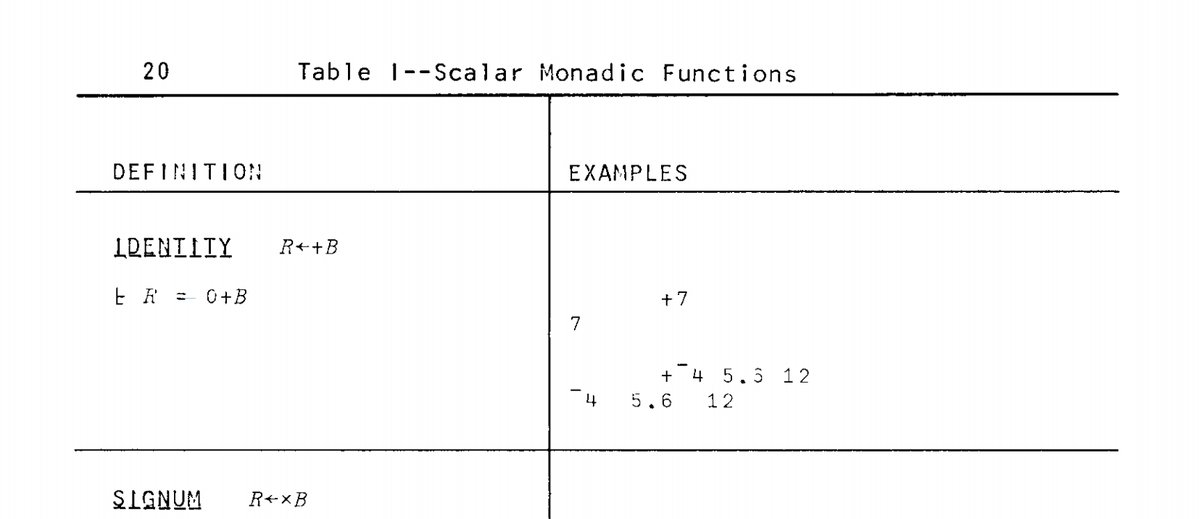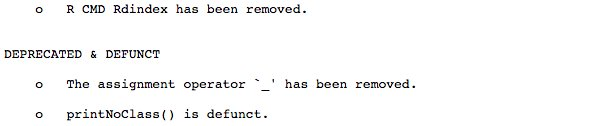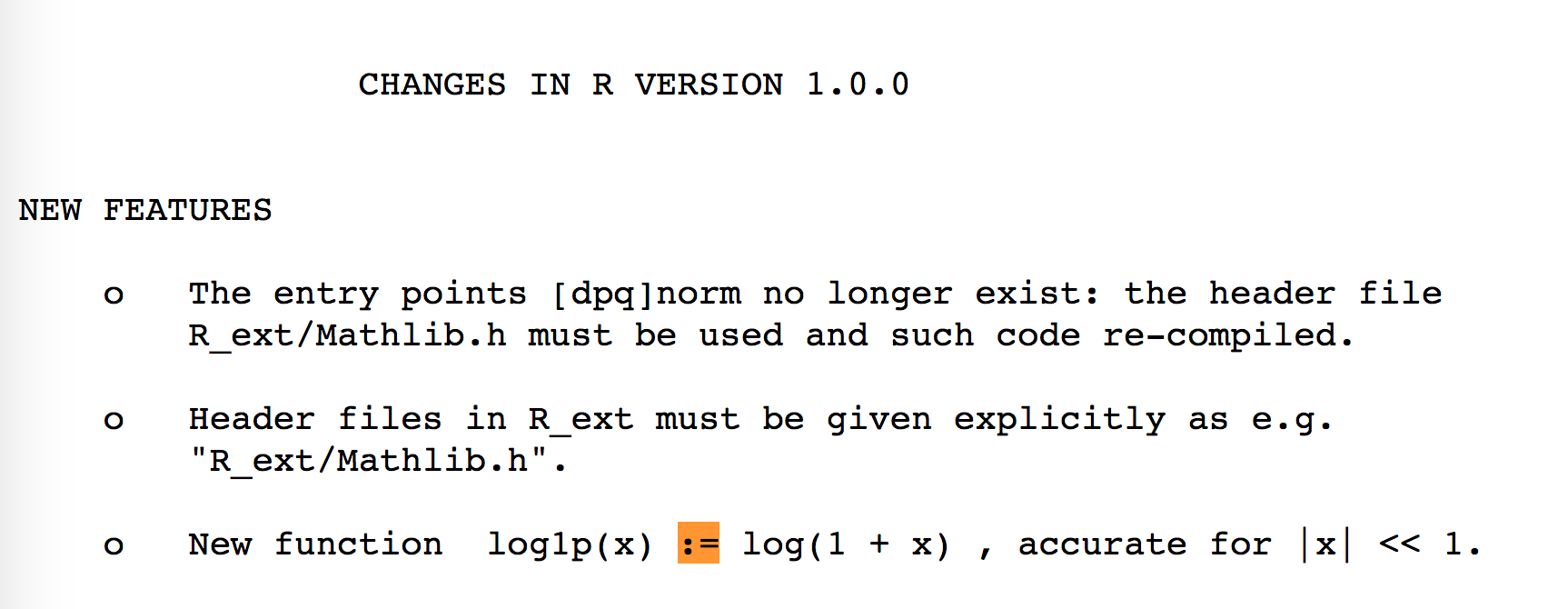Want to share your content on R-bloggers? click here if you have a blog, or here if you don't.

In June, I published a little thread on Twitter about the history of the `<-` assignment operator in R. Here is a blog post version of this thread.

## Historical reasons

As you all know, R comes from S. But you might not know a lot about S (I don’t). This language used `<-` as an assignment operator. It’s partly because it was inspired by a language called APL, which also had this sign for assignment.

But why again? APL was designed on a specific keyboard, which had a key for `<-`:At that time, it was also chosen because there was no `==` for testing equality: equality was tested with `=`, so assigning a variable needed to be done with another symbol.Until 2001, in R, `=` could only be used for assigning function arguments, like ```fun(foo = "bar")``` (remember that R was born in 1993). So before 2001, the `<-` was the standard (and only way) to assign value into a variable.

Before that, `_` was also a valid assignment operator. It was removed in R 1.8:(So no, at that time, no snake_case_naming_convention)

Colin Gillespie published some of his code from early 2000, where assignment was made like this 🙂

The main reason “equal assignment” was introduced is because other languages uses `=` as an assignment method, and because it increased compatibility with S-Plus.

## And today?

Nowadays, there are seldom any cases when you can’t use one in place of the other. It’s safe to use `=` almost everywhere. Yet, `<-` is preferred and advised in R Coding style guides:

One reason, if not historical, to prefer the `<-` is that it clearly states in which side you are making the assignment (you can assign from left to right or from right to left in R):

```a <-  12
13 -> b
a

##  12

b

##  13

a -> b
a <- b
```

The RHS assignment can for example be used for assigning the result of a pipe

```library(dplyr)
iris %>%
filter(Species == "setosa") %>%
select(-Species) %>%
summarise_all(mean) -> res
res

##   Sepal.Length Sepal.Width Petal.Length Petal.Width
## 1        5.006       3.428        1.462       0.246
```

Also, it’s easier to distinguish equality comparison and assignment in the last line of code here:

```c <- 12
d <- 13
e = c == d
f <- c == d
```

Note that `<<-` and `->>` also exist:

```create_plop_pouet <- function(a, b){
plop <<- a
b ->> pouet
}
create_plop_pouet(4, 5)
plop

##  4

pouet

##  5
```

And that Ross Ihaka uses `=` : https://www.stat.auckland.ac.nz/~ihaka/downloads/JSM-2010.pdf

### Environments

There are some environment and precedence differences. For example, assignment with `=` is only done on a functional level, whereas `<-` does it on the top level when called inside as a function argument.

```median(x = 1:10)

##  5.5

x

## Error in eval(expr, envir, enclos): objet 'x' introuvable

median(x <- 1:10)

##  5.5

x

##    1  2  3  4  5  6  7  8  9 10
```

In the first code, you’re passing `x` as the parameter of the `median` function, whereas the second one is creating a variable x in the environment, and uses it as the first argument of `median`. Note that it works because `x` is the name of the parameter of the function, and won’t work with `y`:

```median(y = 12)

## Error in is.factor(x): l'argument "x" est manquant, avec aucune valeur par défaut

median(y <- 12)

##  12
```

There is also a difference in parsing when it comes to both these operators (but I guess this never happens in the real world), one failing and not the other:

```x <- y = 15

## Error in x <- y = 15: impossible de trouver la fonction "<-<-"

x = y <- 15
c(x, y)

##  15 15
```

It is also good practice because it clearly indicates the difference between function arguments and assignation:

```x <- shapiro.test(x = iris\$Sepal.Length)
x

##
##  Shapiro-Wilk normality test
##
## data:  iris\$Sepal.Length
## W = 0.97609, p-value = 0.01018
```

And this weird behavior:

```rm(list = ls())
data.frame(
a = rnorm(10),
b <- rnorm(10)
)

##             a b....rnorm.10.
## 1   0.9885196      1.3809205
## 2  -0.2810080     -1.4165648
## 3  -0.6709831     -1.6203407
## 4  -1.3055656     -1.0713406
## 5   1.2297421      2.2558878
## 6  -1.5333307      0.5194378
## 7  -0.1011028     -0.3651725
## 8  -0.3976268     -1.0814520
## 9  -0.3924576     -0.7030822
## 10 -1.1745994     -0.7090015

a

## Error in eval(expr, envir, enclos): objet 'a' introuvable

b

##    1.3809205 -1.4165648 -1.6203407 -1.0713406  2.2558878  0.5194378
##   -0.3651725 -1.0814520 -0.7030822 -0.7090015
```

## Little bit unrelated but

I love this one:

```g <- 12 -> h
g

##  12

h

##  12
```

Which of course is not doable with `=`.

## Other operators

Some users pointed out on Twitter that this could make the code a little bit harder to read if you come from another language. `<-` is use “only” use in F#, OCaml, R and S (as far as Wikipedia can tell). Even if `<-` is rare in programming, I guess its meaning is quite easy to grasp, though.

Note that the second most used assignment operator is `:=` (`=` being the most common). It’s used in `{data.table}` and `{rlang}` notably. The `:=` operator is not defined in the current R language, but has not been removed, and is still understood by the R parser. You can’t use it on the top level:

```a := 12

## Error in `:=`(a, 12): impossible de trouver la fonction ":="
```

But as it is still understood by the parser, you can use `:=` as an infix without any %%, for assignment, or for anything else:

````:=` <- function(x, y){
x\$y <- NULL
x
}

##   Sepal.Length Sepal.Width Petal.Length Petal.Width Species
## 1          5.1         3.5          1.4         0.2  setosa
## 2          4.9         3.0          1.4         0.2  setosa
## 3          4.7         3.2          1.3         0.2  setosa
## 4          4.6         3.1          1.5         0.2  setosa
## 5          5.0         3.6          1.4         0.2  setosa
## 6          5.4         3.9          1.7         0.4  setosa
```

You can see that `:=` was used as an assignment operator https://developer.r-project.org/equalAssign.html :

All the previously allowed assignment operators (<-, :=, _, and <<-) remain fully in effect

Or in R NEWS 1: